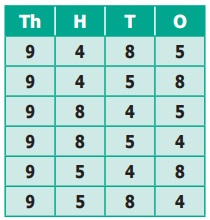Home | | Maths 6th Std | Creating New Numbers

# Creating New Numbers

Using the four digits 9, 4, 8 and 5 we need to make different 4-digit numbers in such a way that the digits are not repeated.

Creating New Numbers

Using the four digits 9, 4, 8 and 5 we need to make different 4-digit numbers in such a way that the digits are not repeated. We get the following arrangement of different 4-digit numbers.Try this

In the same way, try placing the digit 4 in thousands place and get six different 4-digit numbers. Also make different 4-digit numbers by fixing 8 and 5 in the thousands place.Activity

Divide a chart paper into eight equal parts. Write different 1-digit numbers on it. List out the possible 8 digit numbers and also find the largest and the smallest numbers among them.

Tags : Numbers | Term 1 Chapter 1 | 6th Maths , 6th Maths : Term 1 Unit 1 : Numbers
Study Material, Lecturing Notes, Assignment, Reference, Wiki description explanation, brief detail
6th Maths : Term 1 Unit 1 : Numbers : Creating New Numbers | Numbers | Term 1 Chapter 1 | 6th Maths